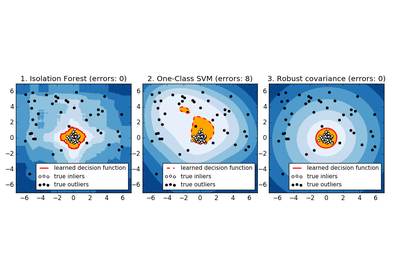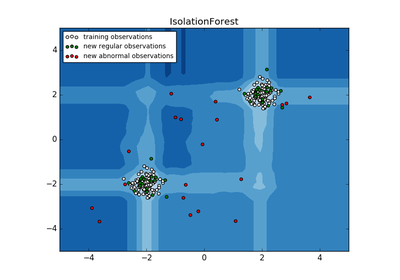# `sklearn.ensemble`.IsolationForest¶

class `sklearn.ensemble.``IsolationForest`(n_estimators=100, max_samples='auto', contamination=0.1, max_features=1.0, bootstrap=False, n_jobs=1, random_state=None, verbose=0)[source]

Isolation Forest Algorithm

Return the anomaly score of each sample using the IsolationForest algorithm

The IsolationForest ‘isolates’ observations by randomly selecting a feature and then randomly selecting a split value between the maximum and minimum values of the selected feature.

Since recursive partitioning can be represented by a tree structure, the number of splittings required to isolate a sample is equivalent to the path length from the root node to the terminating node.

This path length, averaged over a forest of such random trees, is a measure of normality and our decision function.

Random partitioning produces noticeably shorter paths for anomalies. Hence, when a forest of random trees collectively produce shorter path lengths for particular samples, they are highly likely to be anomalies.

Read more in the User Guide.

New in version 0.18.

Parameters: n_estimators : int, optional (default=100) The number of base estimators in the ensemble. max_samples : int or float, optional (default=”auto”) The number of samples to draw from X to train each base estimator. If int, then draw max_samples samples. If float, then draw max_samples * X.shape samples. If “auto”, then max_samples=min(256, n_samples). If max_samples is larger than the number of samples provided, all samples will be used for all trees (no sampling). contamination : float in (0., 0.5), optional (default=0.1) The amount of contamination of the data set, i.e. the proportion of outliers in the data set. Used when fitting to define the threshold on the decision function. max_features : int or float, optional (default=1.0) The number of features to draw from X to train each base estimator. If int, then draw max_features features. If float, then draw max_features * X.shape features. bootstrap : boolean, optional (default=False) If True, individual trees are fit on random subsets of the training data sampled with replacement. If False, sampling without replacement is performed. n_jobs : integer, optional (default=1) The number of jobs to run in parallel for both fit and predict. If -1, then the number of jobs is set to the number of cores. random_state : int, RandomState instance or None, optional (default=None) If int, random_state is the seed used by the random number generator; If RandomState instance, random_state is the random number generator; If None, the random number generator is the RandomState instance used by np.random. verbose : int, optional (default=0) Controls the verbosity of the tree building process. estimators_ : list of DecisionTreeClassifier The collection of fitted sub-estimators. estimators_samples_ : list of arrays The subset of drawn samples (i.e., the in-bag samples) for each base estimator. max_samples_ : integer The actual number of samples

References

 [R161] Liu, Fei Tony, Ting, Kai Ming and Zhou, Zhi-Hua. “Isolation forest.” Data Mining, 2008. ICDM‘08. Eighth IEEE International Conference on.
 [R162] Liu, Fei Tony, Ting, Kai Ming and Zhou, Zhi-Hua. “Isolation-based anomaly detection.” ACM Transactions on Knowledge Discovery from Data (TKDD) 6.1 (2012): 3.

Methods

 `decision_function`(X) Average anomaly score of X of the base classifiers. `fit`(X[, y, sample_weight]) Fit estimator. `get_params`([deep]) Get parameters for this estimator. `predict`(X) Predict if a particular sample is an outlier or not. `set_params`(\*\*params) Set the parameters of this estimator.
`__init__`(n_estimators=100, max_samples='auto', contamination=0.1, max_features=1.0, bootstrap=False, n_jobs=1, random_state=None, verbose=0)[source]
`decision_function`(X)[source]

Average anomaly score of X of the base classifiers.

The anomaly score of an input sample is computed as the mean anomaly score of the trees in the forest.

The measure of normality of an observation given a tree is the depth of the leaf containing this observation, which is equivalent to the number of splittings required to isolate this point. In case of several observations n_left in the leaf, the average path length of a n_left samples isolation tree is added.

Parameters: X : {array-like, sparse matrix}, shape (n_samples, n_features) The training input samples. Sparse matrices are accepted only if they are supported by the base estimator. scores : array of shape (n_samples,) The anomaly score of the input samples. The lower, the more abnormal.
`estimators_samples_`

The subset of drawn samples for each base estimator.

Returns a dynamically generated list of boolean masks identifying the samples used for for fitting each member of the ensemble, i.e., the in-bag samples.

Note: the list is re-created at each call to the property in order to reduce the object memory footprint by not storing the sampling data. Thus fetching the property may be slower than expected.

`fit`(X, y=None, sample_weight=None)[source]

Fit estimator.

Parameters: X : array-like or sparse matrix, shape (n_samples, n_features) The input samples. Use `dtype=np.float32` for maximum efficiency. Sparse matrices are also supported, use sparse `csc_matrix` for maximum efficiency. self : object Returns self.
`get_params`(deep=True)[source]

Get parameters for this estimator.

Parameters: deep : boolean, optional If True, will return the parameters for this estimator and contained subobjects that are estimators. params : mapping of string to any Parameter names mapped to their values.
`predict`(X)[source]

Predict if a particular sample is an outlier or not.

Parameters: X : array-like or sparse matrix, shape (n_samples, n_features) The input samples. Internally, it will be converted to `dtype=np.float32` and if a sparse matrix is provided to a sparse `csr_matrix`. is_inlier : array, shape (n_samples,) For each observations, tells whether or not (+1 or -1) it should be considered as an inlier according to the fitted model.
`set_params`(**params)[source]

Set the parameters of this estimator.

The method works on simple estimators as well as on nested objects (such as pipelines). The latter have parameters of the form `<component>__<parameter>` so that it’s possible to update each component of a nested object.

Returns: self :

## Examples using `sklearn.ensemble.IsolationForest`¶Outlier detection with several methods.IsolationForest example